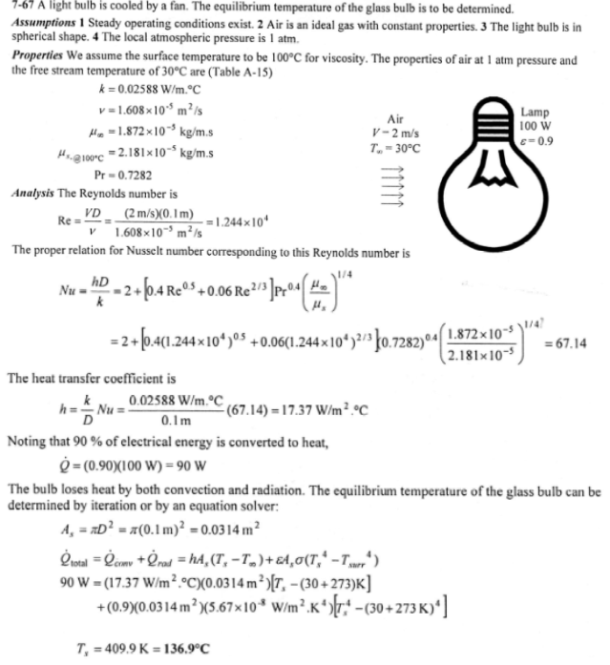heat and mass transfer problems engineering equations heat and mass transfer school homework engineering heat and mass transfer formulas heat and mass transfer solutions to heat and mass transfer problems full solution engineering problem solution heat and mass transfer math problems engineering equations heat and mass transfer school homework engineering solutions to heat and mass transfer formulas heat problems mass problem solutions to transfer problems full solution heat and mass transfer
heat and mass transfer problems engineering equations heat and mass transfer school homework engineering heat and mass transfer formulas heat and mass transfer solutions to heat and mass transfer problems full solution engineering problem solution heat and mass transfer math problems engineering equations heat and mass transfer school homework engineering solutions to heat and mass transfer formulas heat problems mass problem solutions to transfer problems full solution heat and mass transfer
Highalphabet Home Page heat and mass transfer problem solutions Heat and Mass Transfer Page
An incandescent lightbulb is an inexpensive but highly inefficient device that converts electrical energy into light. It converts about 10 percent of the electrical energy it consumes into light while converting the remaining 90 percent into heat. Consider a 10-cm-diameter 100-W lightbulb cooled by a fan that blows air at 30 C to the bulb at a velocity of 2 m/s. The surrounding surfaces are also at 30 C, and the emissivity of the glass is 0.9. Assuming 10 percent of the energy passes through the glass bulb as light with negligible absorption and the rest of the energy is absorbed and dissipated by the bulb itself, determine the equilibrium temperature of the glass bulb.An incandescent lightbulb is an inexpensive but highly inefficient device that converts electrical energy into light. It converts about 10 percent of the electrical energy it consumes into light while converting the remaining 90 percent into heat. Consider a 10-cm-diameter 100-W lightbulb cooled by a fan that blows air at 30 C to the bulb at a velocity of 2 m/s. The surrounding surfaces are also at 30 C, and the emissivity of the glass is 0.9. Assuming 10 percent of the energy passes through the glass bulb as light with negligible absorption and the rest of the energy is absorbed and dissipated by the bulb itself, determine the equilibrium temperature of the glass bulb.